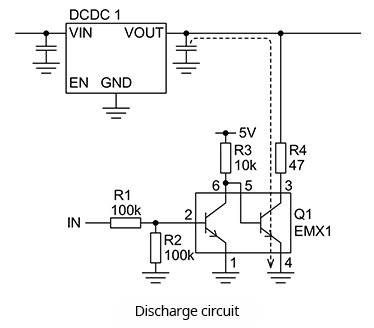# Power Supply Sequence Specification②: Example of Actual Circuit and Component Value Calculations

2023.02.08

・A circuit to realize the power supply sequence ② consists of 3 DCDCs, 2 Power Good blocks, and 3 discharge circuits.

In relation to the Power Supply Sequence Specification ②, in the article before the last and in the previous article, sequence operations upon power turn-on and shutoff were explained. In this article, an actual circuit example to realize the specification ② as well as component value calculations for circuit settings are explained.

## Power Supply Sequence Specification ②： Example of Actual Circuit and Component Value Calculations

Below is shown an example of a circuit that realizes the power supply sequence ②. For the three DCDC systems 1 to 3, switching regulators or linear regulators (LDOs) are assumed. Each DCDC has an enable pin (EN) that can turn the output on and off.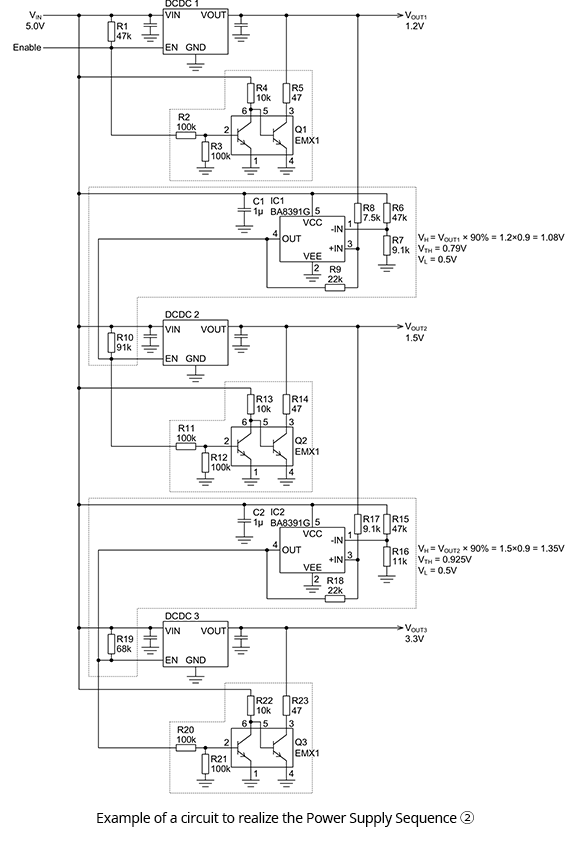### ●Power Good blocks

There are two Power Good blocks. In specification ①, a Power Good function was implemented using a BD4142HFV, which is an IC for voltage monitoring; but in this specification, the Power Good function is configured using the non-inverting hysteresis comparator shown below. In this case, a BA8391G comparator IC is used.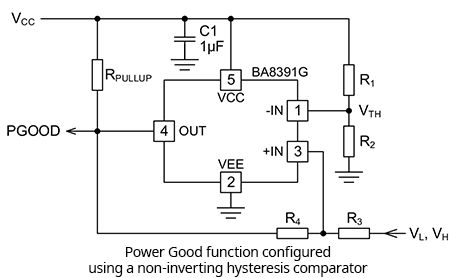A large hysteresis voltage is provided between the detection voltage at startup VH and the detection voltage at shutoff VL. By this means, a single device can detect the voltage at both startup and shutoff and can output a corresponding control signal.

Taking as an example VOUT1 of the above example circuit, we calculate component values. VOUT1 is 1.2 V, and PGOOD for IC1 is set to output a flag upon reaching 90% of the output voltage. The detection voltage at startup VH is 1.2 V×0.9 = 1.08 V. The detection voltage at shutoff VL is set to 0.5 V. These voltages essentially ensure that, even if a reverse voltage is applied across the power supplies, a parasitic element is not turned on.

The comparator threshold voltage VTH is set to midway between VH and VL, as shown below. The value can be determined using equation 2-1.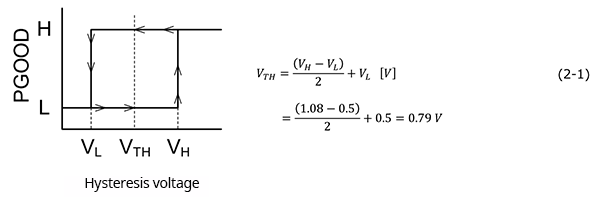VTH can also be expressed as in equation 2-2. Modifying equation 2-2 so as to solve for R2 results in equation 2-3.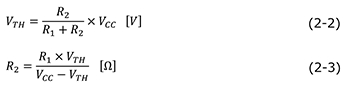With R1 set to 47 kΩ, VCC is supplied from VIN and so is 5 V, and R2 is found from the following equation to be 8.8 kΩ. From the E24 series, a nominal resistance value of 9.1 kΩ is selected.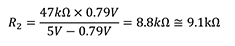R3 is selected so as to be the same as the impedance of the non-inverting input pin (-IN) in order to cancel the input bias current. From equation 2-4, the value is 7.6 kΩ, and from the E24 series, a nominal resistance value of 7.5 kΩ is selected.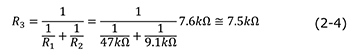Equations 2-5 and 2-6 are general calculation equations to find VH and VL for a non-inverting hysteresis comparator. Upon converting these into equations to find R4 and RPULLUP, we obtain equations 2-7 and 2-8.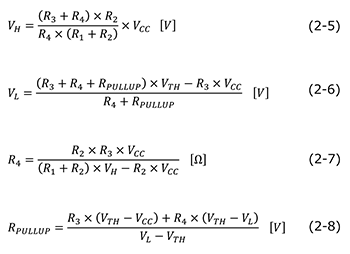Substituting the above-determined component values into equations 2-7 and 2-8, we determine the remaining values.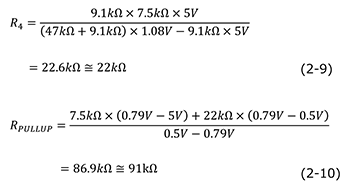A similar procedure can be used for the VOUT2 Power Good block. Please refer to the overall circuit example presented at the beginning.

### ●Discharge circuit

Discretely configured discharge circuits are connected to the DCDCs. As indicated by the diagram below, the circuits consist of NPN transistors and resistors. The first-stage transistor is a simple inverter circuit; the second stage is an open-collector switch. Turn-on of the second stage causes the remaining charge, mainly in the output capacitor, to be discharged at DCDC shutoff, rapidly causing VOUT to fall. The fall time of the output voltage is adjusted through the value of the resistor (R4 in the diagram below) series-connected to the collector of the second-stage transistor. This is the same as in specification ①.